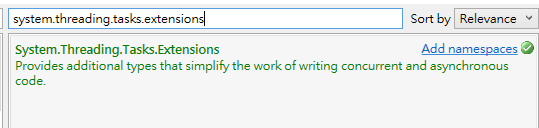var msgs=new List<string>();
foreach (var msg in msgs)
{
await SomeMethodAsync();
//do something
}

return "Hello";
}var msgs=new List<string>();
foreach (var msg in msgs)
{
await SomeMethodAsync();
//do something
}

return "Hello";
}

int res = CalculateSum(0, 0).Result;
Console.WriteLine(res);

async Task<int> CalculateSum(int a, int b)
{
if (a == 0 && b == 0)
{
return 0;
}

return await Task.Run(() => a + b);
}

int res = CalculateSum(0, 0).Result;
Console.WriteLine(res);

async ValueTask<int> CalculateSum(int a, int b)
{
if (a == 0 && b == 0)
{
return 0;
}

return await Task.Run(() => a + b);
}